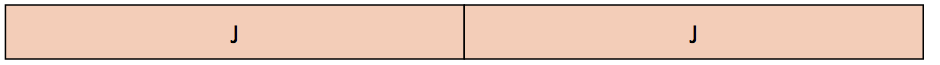Unit Iteration

My Definition

Key Characteristics

The following are true of unit iteration:

• Unit iteration refers to the use of a given unit several times to measure another unit.

• Unit iteration of a unit fraction, such as iterating
1 4
to create
3 4
, represents the relationship between a numerator as a count and a denominator as what is being counted.

• In higher grades, students will see iteration with sequences and numerical approximation.

Example

Use the link below to further study examples of unit iteration.

Unit Iteration Activity

Non-example

If two people share a stick of clay equally, which piece best represents how much of a stick of clay each person will have?Unit J cannot be a unit fraction that is iterated to make a whole. Two units extend past the stick of clay being measured.TEKS: This term is not explicitly covered in the Mathematics TEKS.